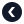Python专题Python 递归

2021-07-19

递归的例子

f(n) = 1 (当n<=2时)
f(n) = f(n-1) + f(n-2) (当n>2时)

def fib(n):
if n<=2:
return 1
else:
return fib(n-1)+fib(n-2)

如何写递归函数

def f(n):
if n<=2:
return 1
else:
return f(n-1)+f(n-2)

递归的优劣

• 递归表达逻辑更加清晰。
• 对编写和维护代码更为友好。 缺点:
• 需要使用更多内存维护堆栈，递归深度过程会有栈溢出的风险。
• 更多的调用栈操作，执行速度会比迭代慢一些。
• 递归中有较多的重复计算，(可以优化)

对递归的优化

重复计算，用缓存

def fib(n):
cache = {}
def innerFib(n):
nonlocal cache
if n<1:
return 0
if n<=2:
return 1
else:
if(n in cache):
return cache[n]
else:
cache[n] = innerFib(n-1)+innerFib(n-2)
return cache[n]

innerFib中定义了一行nonlocal cache，用于声明在innerFib中绑定fib中的cache。

小实验

• fib()是没有加缓存的版本
• fibCache()是带缓存版本的 我们看看两个版本同时计算到 fib(40) 所需要的时间：

堆栈溢出

def fib(n):
if(n<=2):
return 1
a = 1
b = 1
for i in range(3,n+1):
result = a + b
a = b
b = result
return b